# Square Root of Two is Irrational

Square Root of Two is Irrational
Go back to  'Irrational Numbers'

Geometrically, the square root of 2 is the length of the diagonal across a square with sides of one unit of length.

This follows from the Pythagoras Theorem.

It was probably the first number known to be known as irrational.

As a good rational approximation for the square root of two, with a reasonably small denominator, the fraction $$\dfrac{99}{70}$$ is sometimes used.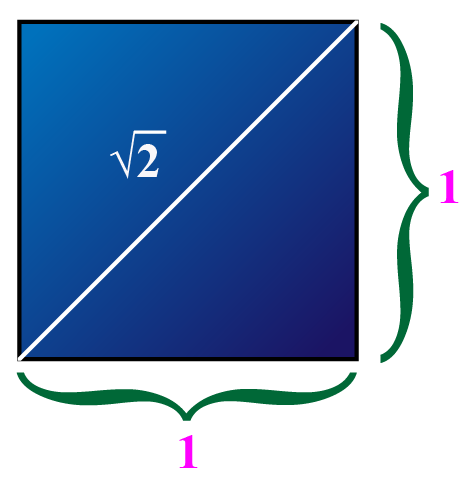Square root of 2 is the diagonal of a unit square.

The square root of 2 is written in mathematics as $$\sqrt{2}$$ or $$2^{\frac{1}{2}}$$

It is a one-half power of 2.

In this mini-lesson, we will explore that the square root of 2 is irrational. We will try to prove that square root of 2 is irrational, understand how to find square root of 2 by giving the proof by contradiction examples and discover other interesting aspects of it. You can check out the interactive simulations to know more about the lesson and try your hand at solving a few interesting practice questions at the end of the page.

## What Is the Square Root of 2?

The square root of a number is the number that gets multiplied to itself to give the original number.

The square root of 2 is represented as $$\sqrt{2}$$.

This is a number that, when multiplied to itself, gives us the result as 2.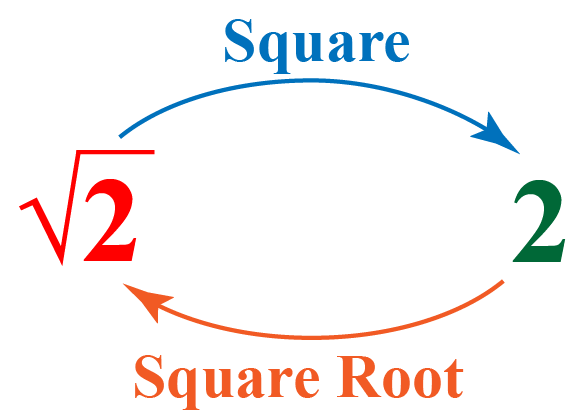## How to Prove That Square Root of 2 Is Irrational?

The actual value of $$\sqrt{2}$$ is undetermined.

The value of $$\sqrt{2}$$ upto 25 decimal places is

 $$\sqrt{2}$$=1.4142135623730950488016887...

At present, the value of $$\sqrt{2}$$ is known up to 1 trillion decimal places.

The decimal expansion of $$\sqrt{2}$$ is infinite because it is non-terminating and non-repeating.

A number with decimal expansion as non-terminating and non-repeating is always an irrational number.

So, $$\sqrt{2}$$ is an irrational number.

Let's prove that square root of 2 is irrational using a contradiction.

Let us assume that $$\sqrt{2}$$ is a rational number.

So, we can write $$\sqrt{2}=\dfrac{p}{q}$$, where $$p$$ and $$q$$ are co-prime numbers and $$q \neq 0$$.

Then, $$\sqrt{2}q=p\;\;\;\;\;\;\cdots (1)$$

Squaring on both sides of the equation (1), we get $$2q^2=p^2 \;\;\;\;\;\;\cdots (2)$$

This means $$p^2$$ is an even number.

This follows that $$p$$ is also an even number.

We can write $$p=2a$$, where $$a \in \mathbb{Z}$$.

Substitute $$2a$$ for $$p$$ in equation (2).

\begin{align}2q^2&=p^2\\2q^{2}&=(2a)^{2}\\2q^{2}&=4a^{2}\\q^{2}&=2a^{2}\end{align}

This means $$q^2$$ is an even number.

This follows that $$q$$ is also an even number.

This implies 2 divides $$p$$ and $$q$$ which is a contradiction because we assumed that $$p$$ and $$q$$ are co-prime numbers.

## How to Find Square Root of 2 by Long Division?

The value of the square root of 2 by long division method consists of the following steps:

### Step 1

Find the largest number whose square is less than or equal to the number 2. Take this number as the divisor and the quotient (1 in this case). Divide and write the remainder.

### Step 2

In the quotient, put a decimal point after 1. Bring down two zeroes to the right of the remainder. So, the new dividend is 100.

### Step 3

Double the divisor and enter it with a blank on its right. Guess the largest possible digit to fill the blank which will also become the new digit in the quotient, such that when the new divisor is multiplied to the new quotient the product is less than or equal to the dividend. Divide and write the remainder.

Repeat this process to get the decimal places you want.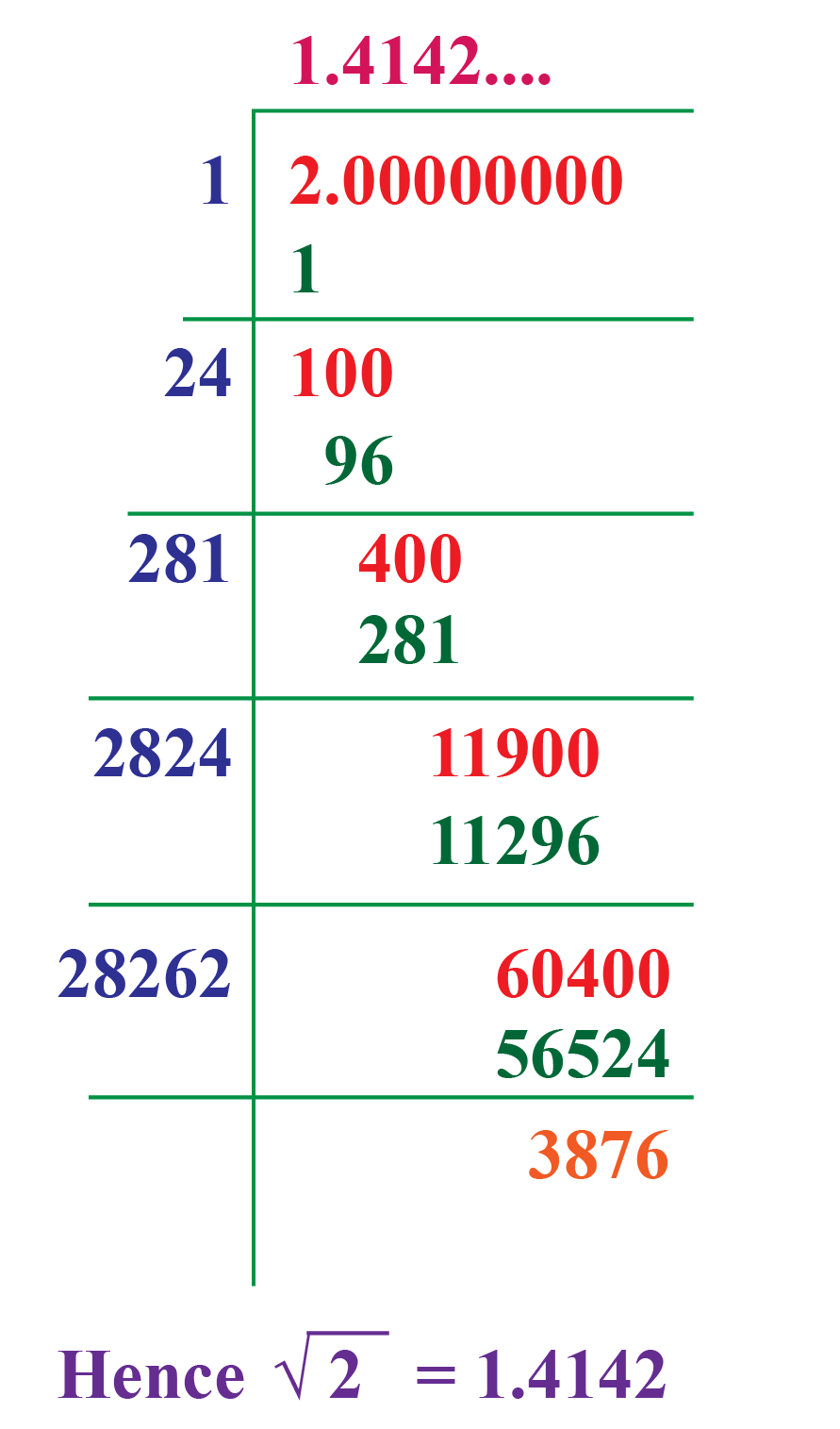Try the simulation below to find the value of $$\sqrt{2}$$.

Drag the slider to set the number of digits after the decimal point you want to calculate in the value of $$\sqrt{2}$$.

## Solved Examples

 Example 1

Tim wants to prove that $$\sqrt{32}$$ is an irrational number.Can you use the fact that the square root of 2 is irrational to prove it?

Solution

We need to find the square root of 32 in terms of the square root of 2.

\begin{align}\sqrt{32}&=\sqrt{2\times2\times2\times2\times2}\\&=2\times2\times\sqrt{2}\\&=4\sqrt{2}\end{align}

Therefore $$\sqrt{32}$$ can also be written as $$4\sqrt{2}$$

Since $$\sqrt{2}$$ is an irrational number, the number $$4\sqrt{2}$$ is also an irrational number.

 $$\therefore$$ $$\sqrt{32}$$ is an irrational number.
 Example 2

Jimmy said her friend Ellyse that the number $$\sqrt{2}$$ is an irrational number.She then asked her to find the square root of 2 using a number line.

Can you help her?

Solution

Let's locate the number $$\sqrt{2}$$ on a number line.

We will use a unit square to discover $$\sqrt{2}$$.

Let's name the vertices of the square as shown.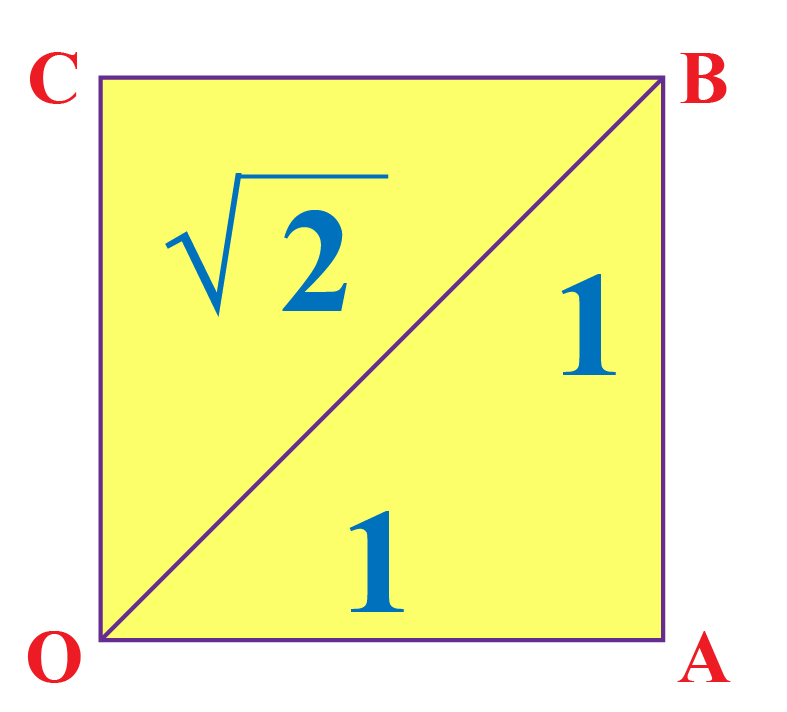Keep the vertex $$O$$ at 0.

We already discovered that $$OB=\sqrt{2}$$

Using a compass with center $$O$$ and radius $$OB$$, draw an arc intersecting the number line at the point $$P$$.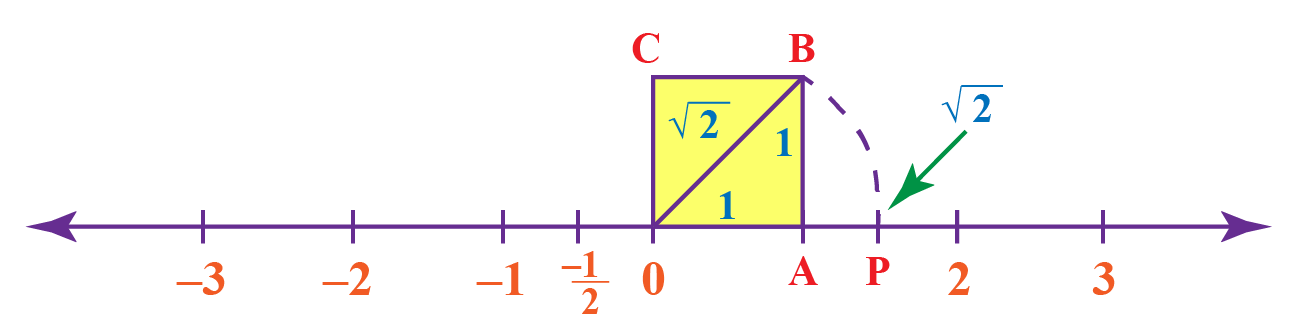$$\therefore$$ The point $$P$$ corresponds to $$\sqrt{2}$$ on the number line.
 Example 3

James is playing a carrom game with his friend Hailey.

The dimensions of the carrom board are $$29\mathrm{\;in}\times29\mathrm{\;in}$$.

Hailey strikes the striker from the diagonal point and by mistake, she pockets the striker at the diagonally opposite hole.

Can you calculate the distance covered by the striker? Is this distance an irrational number?

Solution

The striker covers the diagonal distance of the carrom board.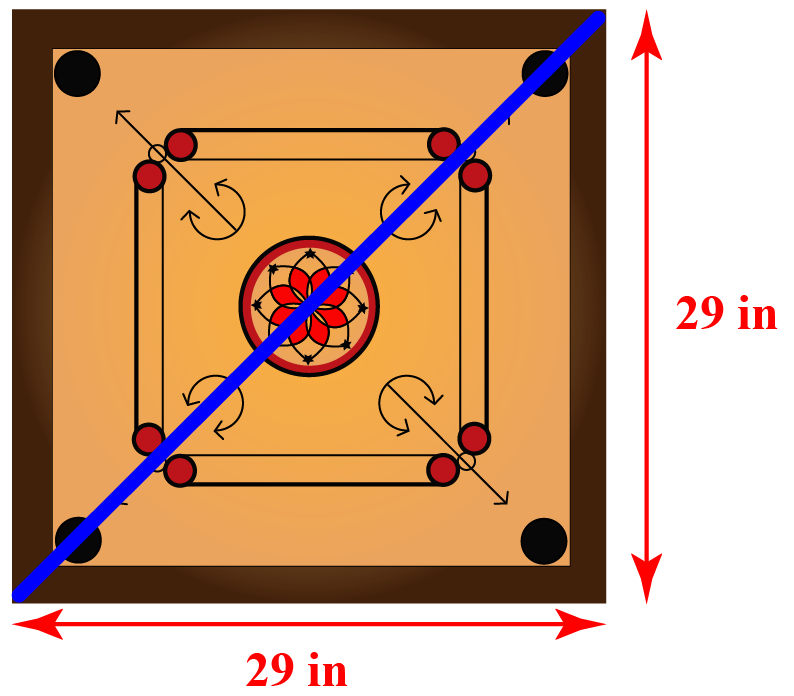Apply the Pythagorean theorem to calculate the distance covered by the striker.

\begin{aligned}\text{Distance covered}&=\sqrt{(29\mathrm{\;in})^2+(29\mathrm{\;in})^2}\\&=\sqrt{2(29)^2\mathrm{\;in}^2}\\&=29\sqrt{2}\mathrm{\;in}\end{aligned}

Therefore the distance covered by the sticker is $$29\sqrt{2}\mathrm{\;in}$$.

Since the number $$\sqrt{2}$$ is an irrational number, the number $$29\sqrt{2}$$ is also an irrational number.

 $$\therefore$$ The distance covered by the striker is $$29\sqrt{2}\mathrm{\;in}$$, and it is an irrational number.Think Tank
1. Can you think of a quadratic equation that has its roots as $$\pm\sqrt{2}$$?
2. Since $$\left(-\sqrt{2}\right)^2=2$$, can we say that $$-\sqrt{2}$$ is also a square root of 2?

## Interactive Questions

Here are a few activities for you to practice.

## Let's Summarize

This mini-lesson targeted the fascinating concept that the square root of 2 is irrational. The math journey around square root of 2 as irrational starts with what a student already knows, and goes on to creatively crafting a fresh concept in the young minds. Done in a way that is not only relatable and easy to grasp but will also stay with them forever. Here lies the magic with Cuemath.

At Cuemath, our team of math experts is dedicated to making learning fun for our favorite readers, the students!

Through an interactive and engaging learning-teaching-learning approach, the teachers explore all angles of a topic.

Be it worksheets, online classes, doubt sessions, or any other form of relation, it’s the logical thinking and smart learning approach that we, at Cuemath, believe in.

## 1. Is the square root of 2 a rational number?

No, the square root of 2 is not a rational number. It is an irrational number.

## 2. What is an irrational number?

A number which can't be expressed in the form $$\dfrac{p}{q}$$.

## 3. What is proof by contradiction?

The proof which is explained using a contradiction is called proof by contradiction.

Numbers and Number Systems
grade 9 | Questions Set 2
Numbers and Number Systems
Numbers and Number Systems
grade 9 | Questions Set 1
Numbers and Number Systems